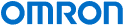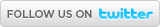Industrial Automation | Europe

# Omron News

## 19/11/2020 - Virtual exhibition showcases the factory of the future13/11/2020 - OMRON awarded platinum rating from EcoVadis for sustainability11/11/2020 - VOLA’s innovative production line with mobile robots wins Danish automation award03/11/2020 - New E3AS-HL Laser Sensor22/10/2020 - Sysmac Studio 3D Simulation boosts productivity# myKnowledge

Main > Product Type > Automation SystemsPrintE-mail
 English Czech Danish Dutch French Finnish German Hungarian Italian Japanese Norwegian Polish Portugese Romanian Russian Spanish Swedish Turkish

# Understanding Floating Point Numbers And Rounding Issues

There are a number of technical issues when using floating point numbers (that is - numbers with a decimal point). These issues are normal and common between all hardware implementations and software applications and are not operation faults:

#### 1) Precision when using any floating point variable

All standard Industry techniques for storage of floating point numbers have some inherent loss of precision or error in storage. There are various types of different sizes. For example 4 bytes, known as "Float" and 8 bytes, known as "Double precision float" or often just "Double". Although these can display a varying number of decimal places this is not the actual accuracy. As the numbers are stored as powers of 2, some decimal numbers cannot be correctly stored. For example the representation of 2.01 is actually 2.00999999999999980

In most cases where floating point numbers of any format are used e.g. when displaying or converting, these precision errors are catered for and the end result is the errors are not normally noticed. However, it is inevitable that in some cases the hidden error becomes visible although in this case, the software is still accurately operating to the limit of its resolution.

An example of this can be seen in CX-Supervisor. If you have 3 text fields on a page as follows

##.##

##.#####

##.##########

If these text fields are linked to a single PLC point that is an IEEE float, when you enter 10.23, you will see the following:

10.23

10.23000

10.2299995422

That is because the most accurate representation of 10.23 that can be stored with IEEE float is 10.229999542236328125. CX-Programmer would display the value as 10.23000 as it is forced to 7 significant digits and the precise value is lost due to display rounding. Sysmac Studio would display the value as 10.23 as it rounds the display to 8 significant digits (10.229999 becomes 10.23). In other cases Sysmac Studio may correctly show all 8 significant digits highlighting the storage error.

Why Floating-Point Numbers May Lose Precision

IEEE Floating Point Converter

What Every Programmer Should Know About Floating-Point Arithmetic

#### 2) Loss of precision when swapping between different floating point lengths

If storing a Double (8 byte) value in a Float (4 byte) value it is inevitable that some information is lost. The Float will store the new value but to less precision e.g. 2.00999999999999980 will become 2.00999998.
A Float (4 byte) value can be stored in a Double (8 byte) value without any loss of precision or change of value however the result may be less precise than the Double would normally be. For example, a float of 2.00999998 converted to a Double is still 2.00999998 which is exactly the same value, but not the same as 2.00999999999999980.

For this reason, floats and doubles should never be used in equality tests e.g. "MyDouble == 2.01" as the loss of precision may cause unexpected results. Instead, always use comparative tests i.e. <, >, >= or <=.

This loss of precision can also be true when performing calculations on floating point numbers, as technically a Float multiplied by a Float could give a Double value although most often the result would be stored in another Float, effectively performing another truncation of precision.

This loss of precision can then affect further use and display as described in (1) above.

#### 3) Loss of precision when using scaling and integer PLC types.

A common problem is when a PLC address stores an integer e.g. is read as "Unsigned Binary Word" or "Unsigned BCD Word" which uses scaling to convert this number into a floating point value.

For example, consider D100, and Unsigned BCD word, storing '0000' to '9999' being scaled to '0' to '999.9'.

This is a perfectly valid example, and may be correct use for some situations. Users will find that if they write a value using 1 decimal place e.g. of 10.1 or 10.2 it works correctly. However, writing values with more decimal places e.g. 10.11, 10.15 or 10.19 results if a different number being returned, from that which was entered (i.e.10.11 becomes 10.1). This can be confusing, but is correct operation.

Exactly the same problem becomes even more confusing with more complex examples, for example consider the same point is scaled to '0' to '599.9'. This means a resolution of 0.06 for each step. Hence, 2.7 would be a valid number (stored as 45 in the PLC), but 2.8 would be rounded to 2.82 (stored as 47 in the PLC). This is exactly the same issue.

In all these cases, the actual number written is rounded to the nearest valid number that can be represented. This is correct operation.